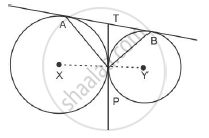# Two circles touch each other externally at P. AB is a common tangent to the circles touching them at A and B. The value of ∠ L APB is - Mathematics

Two circles touch each other externally at P. AB is a common tangent to the circles touching them at A and B. The value of ∠ L APB is

(A) 30°

(B) 45°

(C) 60°

(D) 90°

#### SolutionTA = TP ⇒ ∠TAP = ∠TPA
TB = TP ⇒∠TBP = ´TPB
∠TAP + ∠TBPTA = TP ⇒ ∠TAP = ∠TPA
TB = TP ⇒∠TBP = ´TPB
∠TAP + ∠TBP= ∠TPA + ∠TPB= ∠APB

 ∠TAP + ∠TBP+ ∠APB=180^@[because "sum of" ......180^@]

/_APB+/_APB=180^@

2/_APB=180^@

/_APB=90^@

Concept: Concept of Circle - Centre, Radius, Diameter, Arc, Sector, Chord, Segment, Semicircle, Circumference, Interior and Exterior, Concentric Circles
Is there an error in this question or solution?